# How to Calculate and Solve for Transmissivity | Aquifer Characteristics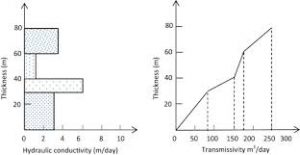The image above represents transmissivity.

To compute for transmissivity, two essential parameters are needed and these parameters are Saturated Thickness of Aquifer (b) and Hydraulic Conductivity (K).

The formula for calculating transmissivity:

T = bK

Where:

T = Transmissivity
b = Saturated Thickness of Aquifer
K = Hydraulic Conductivity

Let’s solve an example;
Find the transmissivity when the saturated thickness of aquifer is 10 and the hydraulic conductivity is 5.

This implies that;

b = Saturated Thickness of Aquifer = 10
K = Hydraulic Conductivity = 5

T = bK
T = 10 x 5
T = 50

Therefore, the transmissivity is 50.

Calculating the Saturated Thickness of Aquifer when the Transmissivity and the Hydraulic Conductivity is Given.

b = T / K

Where;

b = Saturated Thickness of Aquifer
T = Transmissivity
K = Hydraulic Conductivity

Let’s solve an example;
Find the Saturated Thickness of Aquifer when the transmissivity is 28 and the hydraulic conductivity is 7.

This implies that;

T = Transmissivity = 28
K = Hydraulic Conductivity = 7

b = T / K
b = 28 / 7
b = 4

Therefore, the saturated thickness of aquifer is 4.

Calculating the Hydraulic Conductivity when the Transmissivity and the Saturated Thickness of Aquifer is Given.

K = T / b

Where;

K = Hydraulic Conductivity
T = Transmissivity
b = Saturated Thickness of Aquifer

Let’s solve an example;
Find the hydraulic conductivity when the transmissivity is 30 and the saturated thickness of aquifer is 10.

This implies that;

T = Transmissivity = 30
b = Saturated Thickness of Aquifer = 10

K = T / b
K = 30 / 10
K = 3

Therefore, the hydraulic conductivity is 3.

Nickzom Calculator – The Calculator Encyclopedia is capable of calculating the transmissivity.

To get the answer and workings of the transmissivity using the Nickzom Calculator – The Calculator Encyclopedia. First, you need to obtain the app.

You can get this app via any of these means:

To get access to the professional version via web, you need to register and subscribe for NGN 1,500 per annum to have utter access to all functionalities.
You can also try the demo version via https://www.nickzom.org/calculator

Apple (Paid) – https://itunes.apple.com/us/app/nickzom-calculator/id1331162702?mt=8
Once, you have obtained the calculator encyclopedia app, proceed to the Calculator Map, then click on Geology under Add-on.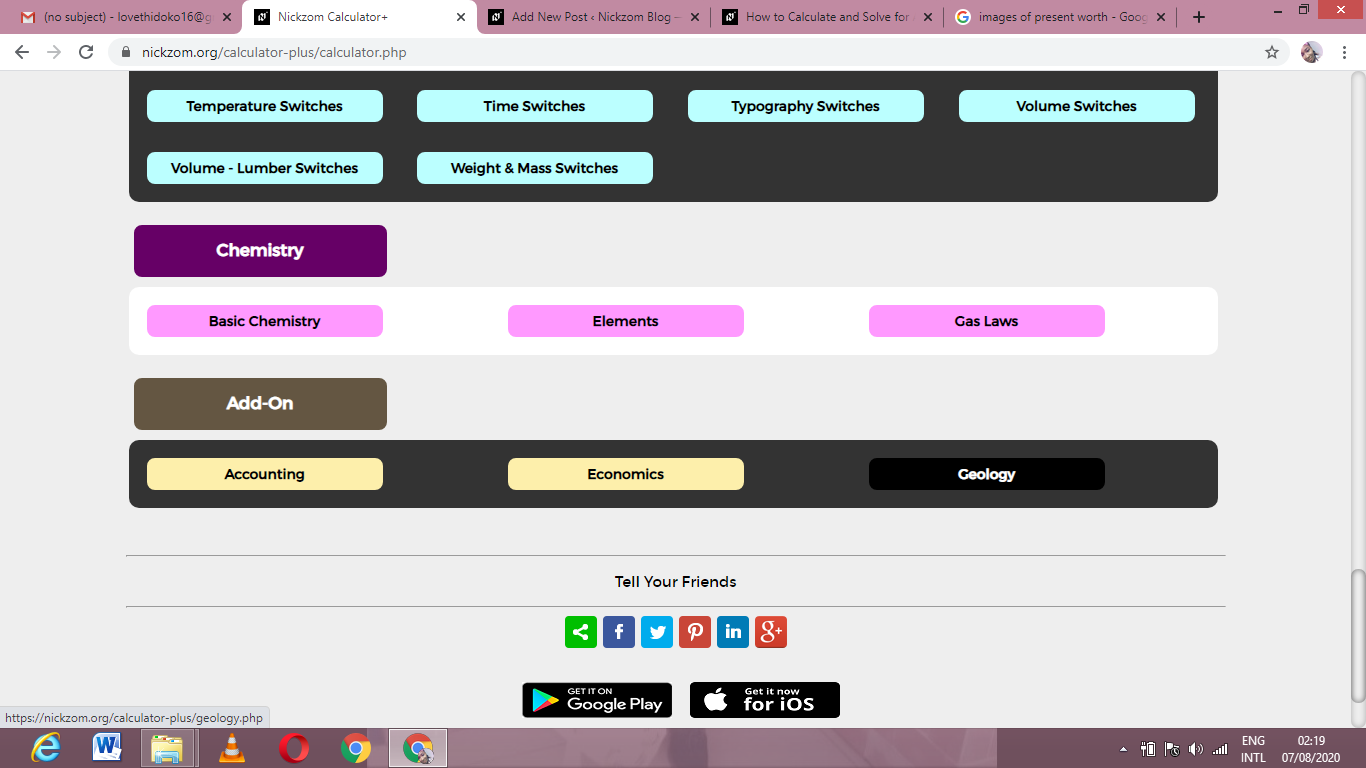Now, Click on Aquifer Characteristics  under Geology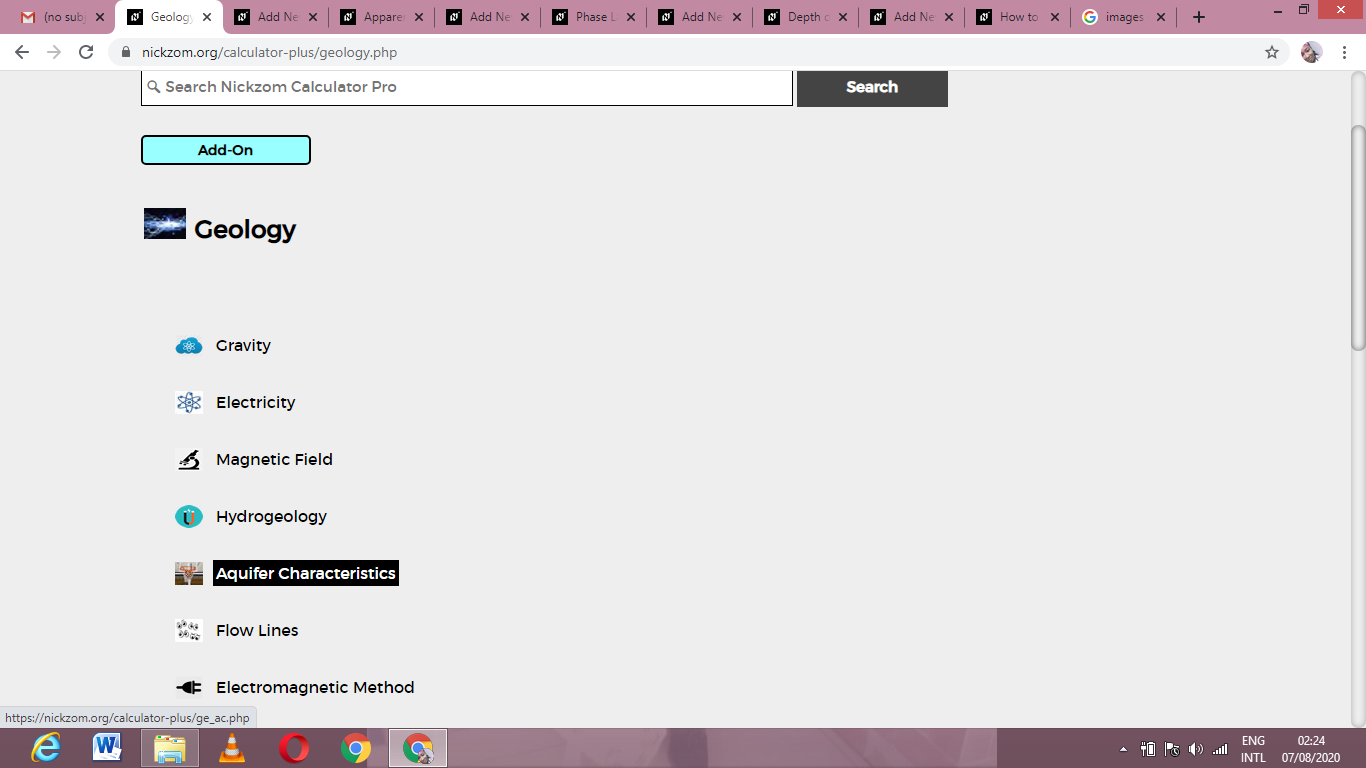Now, Click on Transmissivity under Aquifer Characteristics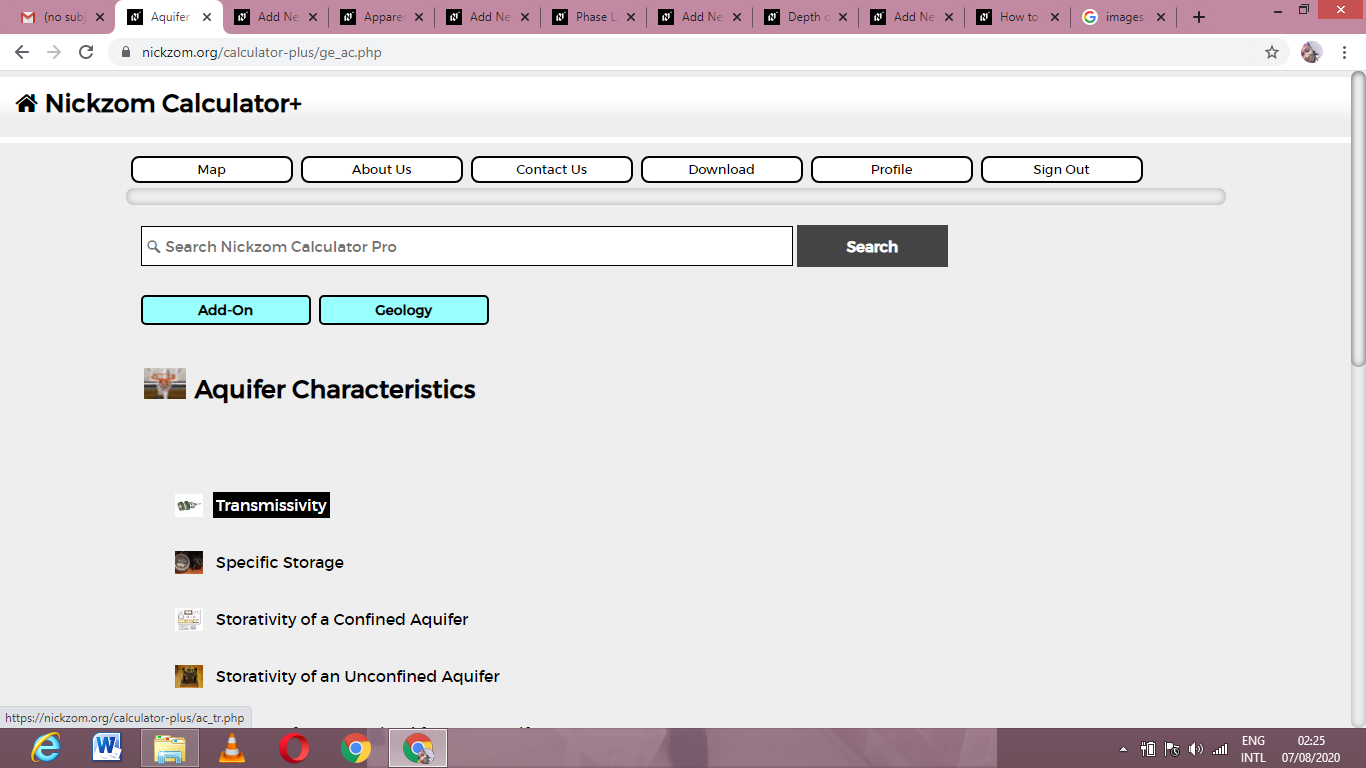The screenshot below displays the page or activity to enter your values, to get the answer for the transmissivity according to the respective parameters which are the Saturated Thickness of Aquifer (b) and Hydraulic Conductivity (K).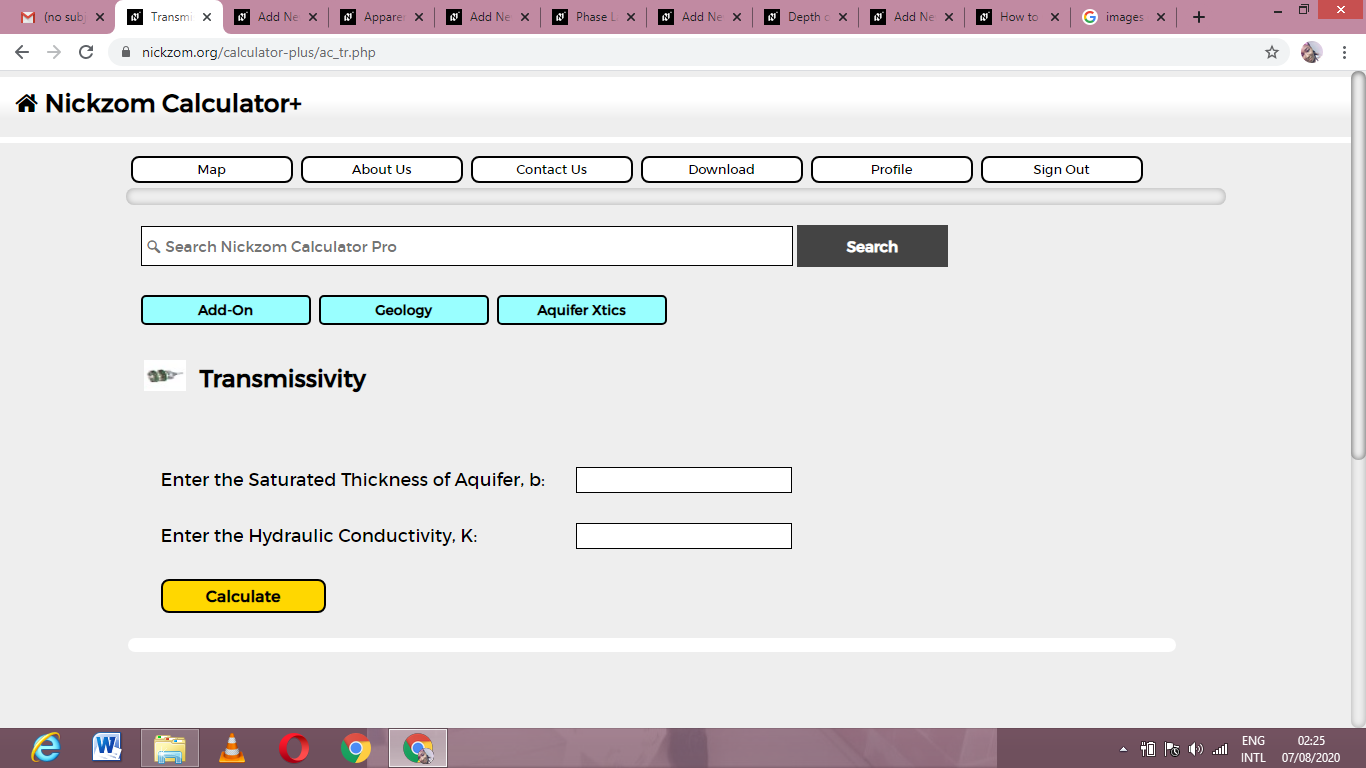Now, enter the values appropriately and accordingly for the parameters as required by the Saturated Thickness of Aquifer (b) is 10 and Hydraulic Conductivity (K) is 5.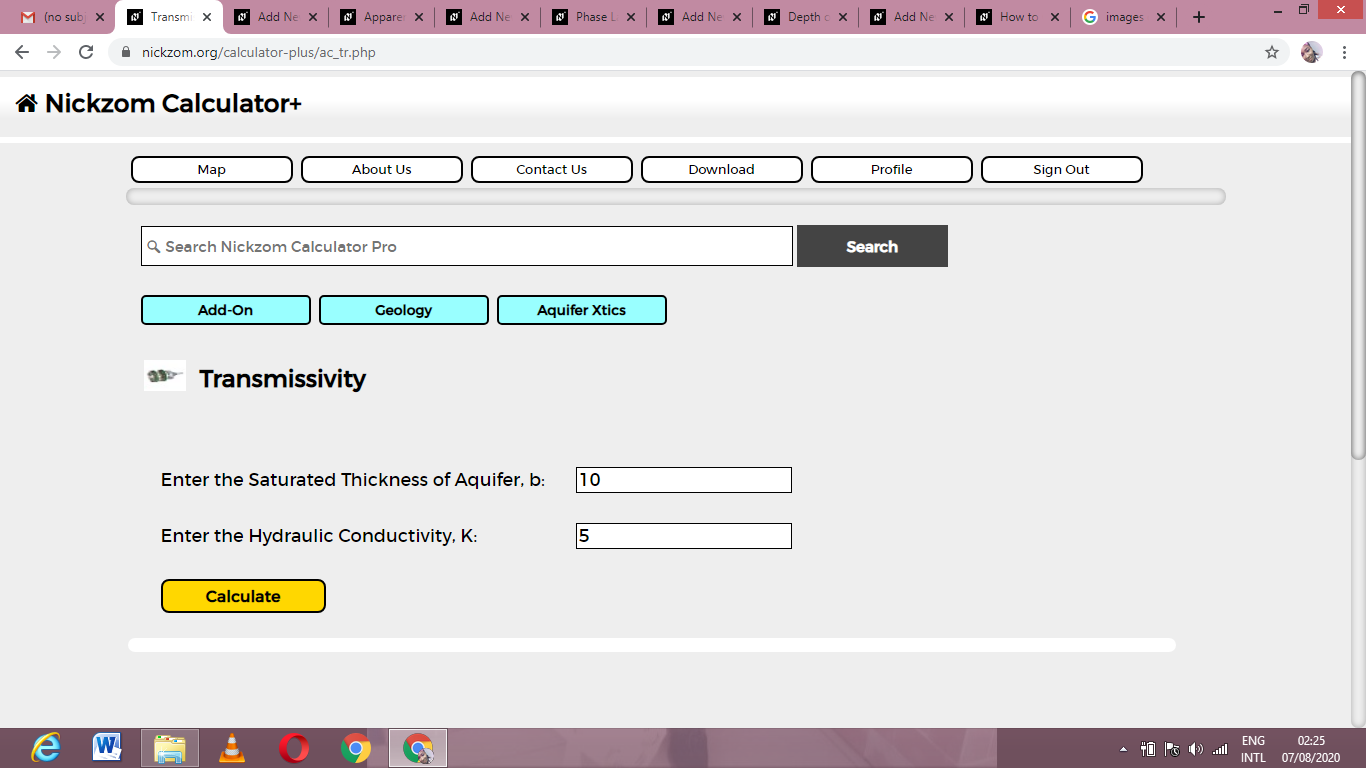Finally, Click on Calculate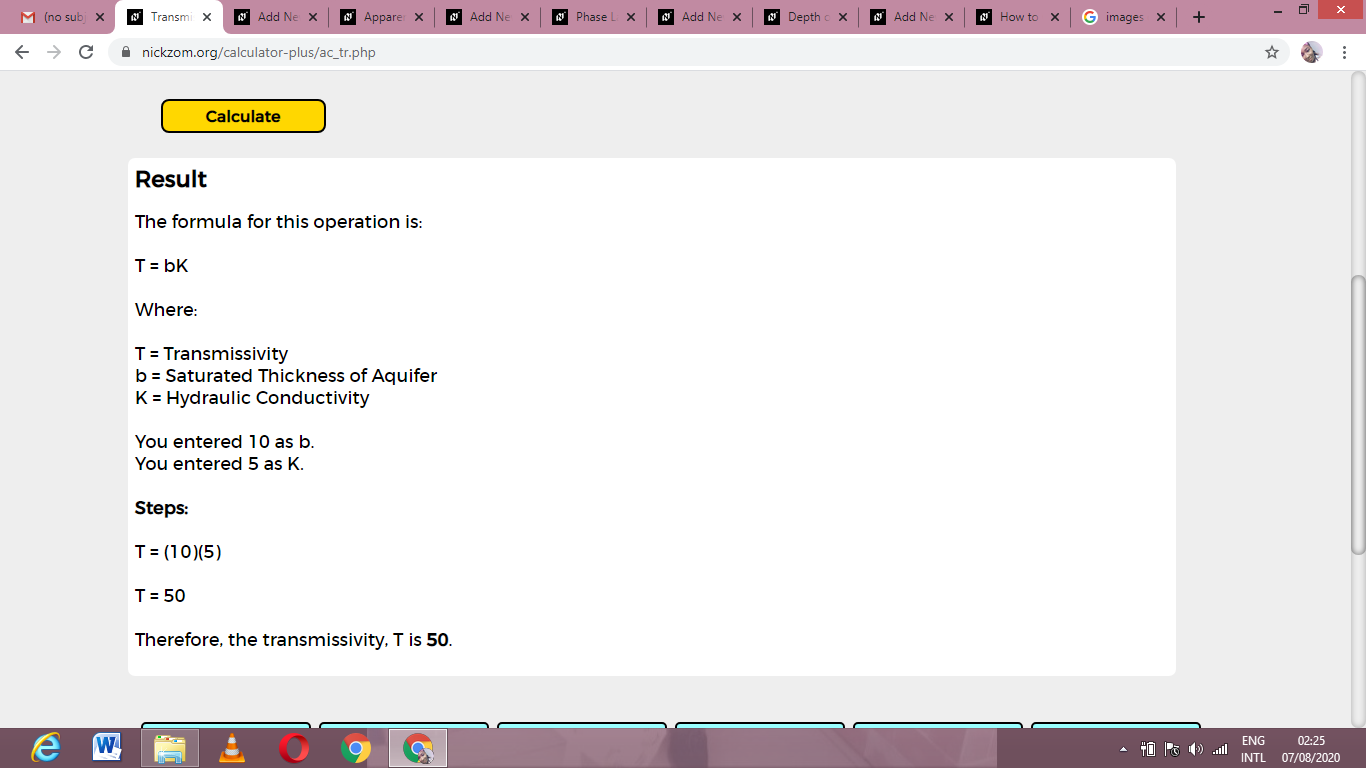As you can see from the screenshot above, Nickzom Calculator– The Calculator Encyclopedia solves for the transmissivity and presents the formula, workings and steps too.